NEET  >  Chemistry: Topic-wise Test- 2

# Chemistry: Topic-wise Test- 2 - NEET

Test Description

## 45 Questions MCQ Test NEET Mock Test Series - Chemistry: Topic-wise Test- 2

Chemistry: Topic-wise Test- 2 for NEET 2023 is part of NEET Mock Test Series preparation. The Chemistry: Topic-wise Test- 2 questions and answers have been prepared according to the NEET exam syllabus.The Chemistry: Topic-wise Test- 2 MCQs are made for NEET 2023 Exam. Find important definitions, questions, notes, meanings, examples, exercises, MCQs and online tests for Chemistry: Topic-wise Test- 2 below.
Solutions of Chemistry: Topic-wise Test- 2 questions in English are available as part of our NEET Mock Test Series for NEET & Chemistry: Topic-wise Test- 2 solutions in Hindi for NEET Mock Test Series course. Download more important topics, notes, lectures and mock test series for NEET Exam by signing up for free. Attempt Chemistry: Topic-wise Test- 2 | 45 questions in 45 minutes | Mock test for NEET preparation | Free important questions MCQ to study NEET Mock Test Series for NEET Exam | Download free PDF with solutions
 1 Crore+ students have signed up on EduRev. Have you?
Chemistry: Topic-wise Test- 2 - Question 1

### Which of the following alkenes will react fastest with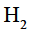under catalytic hydrogenation conditions?

Detailed Solution for Chemistry: Topic-wise Test- 2 - Question 1

The reactivity order towards H2 is
cis > iso > trans i.e in option A the alkene is cis.

Chemistry: Topic-wise Test- 2 - Question 2

### The heat of hydrogenation of 1-hexene is 126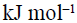. When a second double bond is introduced in the molecule, the heat of hydrogenation of the resulting compound is 230. The resulting compound (diene) is

Detailed Solution for Chemistry: Topic-wise Test- 2 - Question 2

1,3 -hexadiene is a conjugated diene, hence it is more stable than the first compound. The heat of hydrogenation decreases as the stability of the molecule increases. The stability of 13 hexadiene is more than that of a compound which contain two double bonds.

Chemistry: Topic-wise Test- 2 - Question 3

### Heat of hydrogenation of benzene is 51 kcal/mol and its resonance energy is 36 kcal/mol. Then the heats of hydrogenation of cyclohexadiene and cyclohexene per mole respectively are

Detailed Solution for Chemistry: Topic-wise Test- 2 - Question 3

Total energy to break 3 bond in benzene is 87 [51+36].
So to break one bond i.e. in cyclohexaene is 87/3= 29kcal/mol.
And to break 2 bond i.e.in cyclohexadiene is 29*2= 58 kcal/mole.

Chemistry: Topic-wise Test- 2 - Question 4gas is liberated at cathode and anode both by electrolysis of the following aq. solution except in :

Detailed Solution for Chemistry: Topic-wise Test- 2 - Question 4

At the cathode (C), water is reduced to hydroxide and hydrogen gas. The net process is the electrolysis of an aqueous solution of NaCl into industrially

Electrolysis of aqueous sodium chloride yields hydrogen and chlorine, with aqueous sodium. Chlorine gas bubbles out of the melt above the anode.

Chemistry: Topic-wise Test- 2 - Question 5

“10 volume”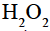means :

Detailed Solution for Chemistry: Topic-wise Test- 2 - Question 5

H2O2 is the special concentration terms.10 volume of H2Omeans that 1ml of H2O2 gives 10 ml of O2.
For example, if it was mentioned 30 volume of H2O2, then its means that 1ml of H2O2 gives 30ml of O2.

Chemistry: Topic-wise Test- 2 - Question 6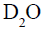(heavy water) and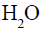differ in following except

Detailed Solution for Chemistry: Topic-wise Test- 2 - Question 6

Chemical properties won't change in case of isotopes. Therefore answer is D.

Chemistry: Topic-wise Test- 2 - Question 7
When 0.532 g of benzene (B.P.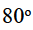C) is burnt in a constant volume system with an excess of oxygen, 22.3 KJ of heat is given out.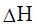for the combustion process is given by :
Chemistry: Topic-wise Test- 2 - Question 8

The work done by the system in a cyclic process involving one mole of an ideal monoatomic gas is  50 KJ/cycle, The heat absorbed by the system per cycle is

Detailed Solution for Chemistry: Topic-wise Test- 2 - Question 8

According to the first law of thermodynamics,
∆Q=∆U+∆W and for a cyclic process, change in internal energy(∆U) is zero. Hence ∆Q=∆W where ∆Q is the heat absorbed and ∆W is the work done. Therefore heat absorbed is 50 kJ

Chemistry: Topic-wise Test- 2 - Question 9

Which of the following is not correct ?

Detailed Solution for Chemistry: Topic-wise Test- 2 - Question 9

It should be equal to T, not (-T)

Chemistry: Topic-wise Test- 2 - Question 10
The heat released when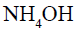& HCl neutralise is :
Chemistry: Topic-wise Test- 2 - Question 11
The heat of formation of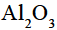is 380 kcals/mole and that of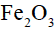is 195 kcals/mole. The heat (in kcals/mole) of the thermite reaction is
Chemistry: Topic-wise Test- 2 - Question 12

Enthalpy of Rhombic sulphur of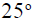C is

Detailed Solution for Chemistry: Topic-wise Test- 2 - Question 12

Rhombic sulphur is the standard state of sulphur or in its pure state.And in standard state it is taken as zero.

Chemistry: Topic-wise Test- 2 - Question 13

Which of the following is not a state function ?

Detailed Solution for Chemistry: Topic-wise Test- 2 - Question 13

A state function is the property of the system whose value depends only on the initial and final state of the system and is independent of the path.Heat (q) and work (W) are not state functions being path dependent.

Chemistry: Topic-wise Test- 2 - Question 14
Which of the following reaction is favored by increase of temperature ?
Detailed Solution for Chemistry: Topic-wise Test- 2 - Question 14
In options a,c and d, we can say that energy is also a part of the products which is indicated by the + sign between the product and the energy but in case of reaction B, you can see that with the products energy is taken inside that is it is a negative sign so when we transfer that part of the equation to the product side, we can see that in the product there is a sign of plus along with energy which means that energy is given along with the products to obtain the reactants .you can conclude that option B is the correct answer because it is an endothermic reaction
Chemistry: Topic-wise Test- 2 - Question 15
The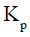for the decomposition of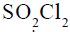(if its degree of dissociation under one atomic pressure is 90%) is
Chemistry: Topic-wise Test- 2 - Question 16

The pH of 10-7 M HCl is

Detailed Solution for Chemistry: Topic-wise Test- 2 - Question 16

Without calculation one can also say that the answer is a because acid has a ph value less than 7.

Chemistry: Topic-wise Test- 2 - Question 17

1c.c. of 0.1N HCl is added to 99 c.c. aqueous solution of sodium chloride.The pH of the resulting solution will be

Detailed Solution for Chemistry: Topic-wise Test- 2 - Question 17

Normality volume = (0.1) (0.001)
Normality volume = 0.0001 L
Volume of solution = Volume of NaCl + Volume of HCL
Volume of solution = 1 + 0.001
Volume of solution = 1.001 L
Since NaCl is a salt and is neutral so it does not effect pH of a solution.
Now,
Normality of HCL in a resulting solution = 0.0001 / 1.001
Normality of HCL in a resulting solution = 0.0001 N
So,
pH = - log [H⁺]
pH = - log (0.0001)
pH = 4
which shows that the pH of the resulting solution is 4

Chemistry: Topic-wise Test- 2 - Question 18

0.2 molar aqueous solution of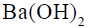is found to be 90% ionized at 298 K.The pH of the solution is

Detailed Solution for Chemistry: Topic-wise Test- 2 - Question 18

Lets calculate the pOH first,

Since 1 mol Ba(OH)2 gives 2 moles of OH- 0.2 moles will give 0.4 mol OH-

However its 90% ionised and hence [OH-] = 0.36 M

So pOH = -log [0.36]

= 0.443

pH = 14-0.443

= 13.55

Chemistry: Topic-wise Test- 2 - Question 19

If the maximum concentration of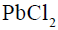in water is 0.01M at 298K, its maximum concentration in 0.1M NaCl will be

Detailed Solution for Chemistry: Topic-wise Test- 2 - Question 19

Concentrations of the ions:

The dissociation reaction for PbCl2​ in water is write as,

PbCl2​→Pb2+(aq)+2Cl−(aq)

The maximum concentration of PbCl2 in water is 0.01 M

We can find concentration of the ions using the stoichiometry from the above reaction.

The concentration of Pb²⁺ ion is 0.01 M and concentration of Cl⁻ ion is 2×0.01=0.02M.

Solubility product:

The solubility product of PbCl2​ can be written as,

KSP​=[Pb2+][Cl]−2

Let us insert in the concentrations of Pb and Cl.

KSP​=(0.01)(0.02)

Ksp =4.0×10−6

Concentration of Pb2+ using common ion effect:
WhenPbCl2​ is dissolved in 1MNaCl, there is a common ion Cl−. This decreases the solubility of PbCl2​ due to the common ion effect.
The concentration of Cl− in 0.1MNaCl is 0.1. Let us plug in this value to find the new concentration of Pb2+ ion.

Ksp=[Pb2+][Cl]2

4×10−6=[Pb2+](0.1)2

[Pb2+]=0.014×10−6​

[Pb2+]=0.0004M=4×10−4 M

Chemistry: Topic-wise Test- 2 - Question 20

When equal volumes of the following solution are mixed, precipitation of AgCl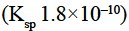will occur only with

Detailed Solution for Chemistry: Topic-wise Test- 2 - Question 20

AgCl is precipitated only when [Ag+][Cl-] > Ksp
On mixing equal volumes of two solutions, concentration becomes halved.Chemistry: Topic-wise Test- 2 - Question 21

Ammonium hydrogen sulphide is contained in a closed vessel at 313 K when total pressure at equilibrium is found to be 0.8 atm. The value offor the reaction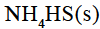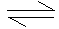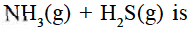Detailed Solution for Chemistry: Topic-wise Test- 2 - Question 21

Ammonium hydrogen sulphide is contained in a closed vessel at 313K when total pressure at equilibrium is found to be 0.8 atm.

NH4​HS(s)⇌NH3​(g)+H2​S(g)

PNH3​​=PH2​S​=P

Total pressure PNH3​​+PH2​S​=P+P=2P

But total pressure is 0.8 atm.

2P=0.8

P=0.4 atm

PNH3​​=PH2​S​=P=0.4 atm

The value of the equilibrium constant for the reaction,is Kp​=PNH3​​×PH2S

Kp​=0.4×0.4

Kp​=0.16

Chemistry: Topic-wise Test- 2 - Question 22

Which of the following hydrocarbons has the highest melting point?

Detailed Solution for Chemistry: Topic-wise Test- 2 - Question 22

Melting point order is para > ortho > meta on basis of symmetry . So option b is correct.

Chemistry: Topic-wise Test- 2 - Question 23
Propylene is passed through conc.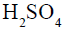and the prodcut thus obtained on hydrolysis with boiling water gives
Chemistry: Topic-wise Test- 2 - Question 24

Reaction of ethene with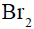in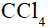gives

Detailed Solution for Chemistry: Topic-wise Test- 2 - Question 24

When alkenes react with Br2 in presence of CCl4 it gives vicinnyl dibromides (1 2 dibromide )

Chemistry: Topic-wise Test- 2 - Question 25

The major product obtained when isobutane is treated with chlorine in the presence of light is

Detailed Solution for Chemistry: Topic-wise Test- 2 - Question 25

The major product is tert butyl chloride as firstly isobutylchloride is formed but then hydride shift takes place due to which chlorine shift to more stable tertiary carbocation and hence the product is tert butyl chloride.

Chemistry: Topic-wise Test- 2 - Question 26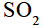acts as

Detailed Solution for Chemistry: Topic-wise Test- 2 - Question 26

SO2 can act as both oxidizing as well as reducing agent since it has +4 oxidation state which is exactly between
its highest oxidation state +6 and lowest oxidation state +2 ,
Thus it can change its oxidation number either from +4 to-2(reduction) and +4 to+6 (oxidation)

Chemistry: Topic-wise Test- 2 - Question 27

Which one of the following leads to a redox reaction

Detailed Solution for Chemistry: Topic-wise Test- 2 - Question 27

Redox reactions are characterized by the transfer of electrons between chemical species, most often with one species undergoing oxidation while another species undergoes reduction.

2KI+Cl2​⟶2KCl +I2​

It is an example of a redox reaction.

Hence, option C is correct.

Chemistry: Topic-wise Test- 2 - Question 28

The compound contains atoms X,Y,Z. The oxidation number of X is 2, Y is 5 and Z is –2, a possible formula of the compound is

Detailed Solution for Chemistry: Topic-wise Test- 2 - Question 28

The net charge on the compound should be zero. Or the sum of oxidation states = 0.

Given: oxidation number of X is +2, Y is +5 and Z is -2.

In XYZ2​:

2+5+2×(−2)=+3

In Y2​(XZ3​)2​:

2×5+2×(2+3×(−2))=+2

In X3​(YZ4​)2​:

3×2+2×(5+4×(−2))=0

In X3​(YZ4​)2​:

3×2+2×(5+4×(−2))=0

In X3​(Y4​Z)2​:

3×2+2×(4×5−2)=42

A compound contains atoms X, Y and Z . The oxidation number of X is +2 , Y is +5 and Z is -2.

The possible formula of the compound is X3​(YZ4​)2​.

Chemistry: Topic-wise Test- 2 - Question 29
Which of the following statements is correct regarding the structures of the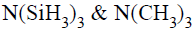molecules
Chemistry: Topic-wise Test- 2 - Question 30

How many allotropic forms of carbon are there ?

Detailed Solution for Chemistry: Topic-wise Test- 2 - Question 30

Carbon has three well known allotropes which are graphite, daimond and buck minster fullerene.

Chemistry: Topic-wise Test- 2 - Question 31

Graphite has a layered structure. The distance between the layers is

Detailed Solution for Chemistry: Topic-wise Test- 2 - Question 31

Since the layers are only weakly attracted to each other, they can easily slide past one another. This is why graphite is used in pencil lead. The distance between layers is 335 pm. Carbon atoms in diamond are covalently bonded and are arranged in a three-dimensional tetrahedral structure.

Chemistry: Topic-wise Test- 2 - Question 32

Coal gas is a mixture of

Detailed Solution for Chemistry: Topic-wise Test- 2 - Question 32

Coal gas contains a variety of calorific gases including hydrogen, carbon monoxide, methane, ethylene and volatile hydrocarbons together with small quantities of non-calorific gases such as carbon dioxide and nitrogen.

Chemistry: Topic-wise Test- 2 - Question 33

Formic acid (HCOOH) on heating with concentratedgives

Detailed Solution for Chemistry: Topic-wise Test- 2 - Question 33

H2SO4 + HCOOH → CO + H2SO4•H2O.
Sulfuric acid react with formic acid to produce carbon monoxide and hydrate sulfuric acid.

Chemistry: Topic-wise Test- 2 - Question 34

Which of the following gases is used for the purification of nickel ?

Detailed Solution for Chemistry: Topic-wise Test- 2 - Question 34

CO is the gas used for purification of nickel and this process is called Mond process.

Chemistry: Topic-wise Test- 2 - Question 35

The most stable oxidation state of boron is

Detailed Solution for Chemistry: Topic-wise Test- 2 - Question 35

The elments of the boron family adopts oxidation states +3 or +1. The +3 oxidation states are favorable except for the heavier elements, such as Tl, which prefer the +1 oxidation state due to its stability; this is known as the inert pair effect.

Chemistry: Topic-wise Test- 2 - Question 36

Which of the following products are formed when boron is fused with NaOH ?

Detailed Solution for Chemistry: Topic-wise Test- 2 - Question 36

Boron reacts with fused sodium hydroxide to form sodium borate and hydrogen.
B + 6NaOH →→ 2Na3BO3 + 3H2

Chemistry: Topic-wise Test- 2 - Question 37

When borax is dissolved in water,

Chemistry: Topic-wise Test- 2 - Question 38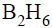reacts with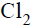to produce

Detailed Solution for Chemistry: Topic-wise Test- 2 - Question 38

B2H6 + 6Cl2 → 2BCl3 + 6HCl

Diborane react with chlorine to produce boron(III) chloride and hydrogen chloride...

Chemistry: Topic-wise Test- 2 - Question 39

In the Serepeck process, the bauxite ore is treated with

Detailed Solution for Chemistry: Topic-wise Test- 2 - Question 39

Serpeck process is a method of purification of Bauxite ore containing silica (SiO2​) as the main impurity.

The reaction involved are-

Al2​O3​.2H2​O+3C+N2​⟶2AlN+3CO+2H2​O

2AlN+6H2​O⟶2NH3​+Al(OH)3

Chemistry: Topic-wise Test- 2 - Question 40

Anhydrous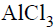produces fumes in the air because of

Detailed Solution for Chemistry: Topic-wise Test- 2 - Question 40

Anhydrous aluminium chloride is hydrolised partly with the moisture in the atmosphere to give HCl gas. This HCl gets combine to moisture in air giving white fumes. So white fumes appear by hydrolysis.

Chemistry: Topic-wise Test- 2 - Question 41

Alzeimer’s diseases is caused by the depostion of

Chemistry: Topic-wise Test- 2 - Question 42

A real gas obeying van der Waals equation will resemble an ideal gas if

Detailed Solution for Chemistry: Topic-wise Test- 2 - Question 42

According to van der Waals equation for 1 mole of a real gas
(P+(a/V2)​)(V−b)=RT
If a and b are small, a/V2 and b can be neglected as compared to P and V and the equation reduces to PV=RT. Hence, a real gas will resemble an ideal gas when constants a and b are small.

Chemistry: Topic-wise Test- 2 - Question 43

In which of the following pairs do the molecules (gaseous) have the same root-mean-square speed

Detailed Solution for Chemistry: Topic-wise Test- 2 - Question 43

RMS speed = √(3RT/M) where, T is temperature, R is universal gas constant and M is molecular mass of gas
Here molecular mass is same for N2 (14x2=28) and CO (12+16=28) so rms speed will also b same for them as rest all is constant

Chemistry: Topic-wise Test- 2 - Question 44
According to the kinetic theory of gases, between two successive collisions, an ideal-gas molecule travels
Detailed Solution for Chemistry: Topic-wise Test- 2 - Question 44
According to kinetic theory the gas molecules are in a state of constant rapid motion in all possible directions colloiding in a random manner with one another and with the walls of the container and between two successive collisions molecules travel in a straight line path but show haphazard motion due to collisions.
Chemistry: Topic-wise Test- 2 - Question 45

The pressure at the base of a column of liquid of length l and held at an angle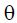to the vertical is

Detailed Solution for Chemistry: Topic-wise Test- 2 - Question 45

Pressure at the base of a liquid column =hdg

Here h=height of the column

d=density of liquid

g=acceleration due to gravity

since the column is inclined at an angle θ the vertical height=l cosθ

Pressure=lcosθ*d*g

=ldgcosθ

Here P=d=density

so option (d) is correct

=pgl cosθ

## NEET Mock Test Series

1 videos|24 docs|145 tests
Information about Chemistry: Topic-wise Test- 2 Page
In this test you can find the Exam questions for Chemistry: Topic-wise Test- 2 solved & explained in the simplest way possible. Besides giving Questions and answers for Chemistry: Topic-wise Test- 2, EduRev gives you an ample number of Online tests for practice

## NEET Mock Test Series

1 videos|24 docs|145 tests

### How to Prepare for NEET

Read our guide to prepare for NEET which is created by Toppers & the best Teachers